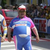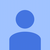0
Planned

# Speed Comparison to MATLAB Double Precision

Sarcastic Processor 5 years ago updated by Pavel Holoborodko 4 years ago
I see speed comparisons vs. MAPLE. Do you have speed comparisons (slowdown factor) vs. MATLAB double precision? Thanks.Under review
Toolbox is not meant to replace the double precision computations.

Extended precision provided in toolbox is targeted to solve problems, which are not solvable in double precision (sensitive eigenvalues, ill-conditioned systems, etc.).

What comparison is possible here ;)? Solved / Not solved?

Speed is irrelevant if problem is not solved, right?Pavel, you have misinterpreted my intent. Rather than the mindset of showing performance comparisons to MAPLE's arbitrary precision as demonstrating superiority to the competition, I am interested in an idea of what the computational time to be expected using the Multiprecision Computing Toolbox is, with MATLAB double precision as a well-known comparison point. It would be useful to understand how computation speed in Multiprecision Computing Toolbox varies with the precision selected. One of these precisions, so to speak, would be MATLAB double precision.

So ideally, a computation time table, with columns for MATLAB double precision, and Multiprecision Computing Toolbox in various precisions. And if it is the case that quad precision is the fastest multiprecision precision, then certainly those results should be part of such a table, and to me, would be the most important column.

Thanks.One small update. In some special cases, quad precision in toolbox is now faster than MATLAB's double:Sorry for misunderstanding.

It is good idea to measure toolbox timings for different level of precision (actually we do this internally, but don't publish as our website is already over-filled with tables). But again, only for the case of extended precision, not double.

I think, in the world of arbitrary precision software we need to use different "well-known comparison point".
Probably toolbox might be the one since now it is the fastest among 3M (Maple, Matlab and Mathematica) :).

Double precision is implemented in hardware, extended in software. Slowdown factor might be as high as 100-1000.Can you provide guidance for various computations on slowdown factor for quad precision vs. MATLAB double precision? Is quad precision in fact the precision with the least slowdown?Sorry I don't collect such comparison. Yes, quadruple has the lowest slowdown.

I think the fastest way would be to just compare particular algorithm(s) of interest.
Toolbox trial is full functional and has all the optimizations - it can be downloaded from our website and run in a few minutes.Hi.

Most computations take around 20 times longer in mp quad precision compared to Matlab double precision, which is already quite good. It can go up to 300 times if Matlab uses a well parallelized algorithm compared to mp like matrix multiplication of big matrices. quad precision ( I use mp.digits(34), mp.GuardDigits(0)) is defenetly the fastest compared to any other, even lower, precision. mp defently outperformes vpa in any case.Matlab's multiplication uses hardware SIMD vectorization. Something we cannot employ for extended precision :(.
This makes all the difference (mtimes is also parallelized in toolbox).Michael_

Thank you. That is very helpful information. The insight about the effect of parallelization is particularly informative. Unfortunately for me, the only calculations for which I really care about computation time are matrix calculations on large matrices. That doesn't mean I'm not willing to use quad precision when needed, but if I have a calculation that takes a day to run in MATLAB double precision, I will assess the almost year it would take in quad precision to be unviable. Heck, even 3 weeks probably isn't going to cut it. On the other hand, I have some situations where I think there is just s small part of a large algorithm where I need to perform quad precision (dealing with matrices which can get very ill-conditioned), while retaining most of the most computationally intensive portions in MATLAB double precision.

I wish the powers that be had moved to hardware level support for quad precision, but alas, with the popularity of GPUs, often run in single precision, the world seems to be heading in the other direction. Of course that would put Pavel out of business, but I guess he could implement an octuple precision to keep it going.Actually for big matrices extended precision might be faster than double in some cases. For example, Krylov-type solvers CG, GMRES, etc. suffers from inherent loss of orthogonality in subspace basis with iterations - which leads to very slow convergence and the need for restarts, double application of modified Gram-Schmidt, etc.

Extended precision makes all this disappear and can actually guarantee theoretical convergence speed e.g. for CG (which is never seen in practice for double precision if no good pre-conditioner is known).

Precisions < 50 are already pretty much comparable to the speed of quadruple. So that we want to avoid strong division on quadruple, octuple, etc.

We also have plans to implement (at least) quadruple on GPU - prototype engine is tested and ready for implementation. The only question is time and development funding, as always.PlannedPavel,

Immediately above, you made a post consisting of just PLANNED in a blue rectangle. Can you please elaborate on what is planned, or what your post means? Thanks.Hello,

I was just organizing the forum. Original question was in "Under review" status. I changed it to "Planned" to reflect that I already handled it and took your advice into consideration - will pay more attention to comparison with "double" in future.

(Software used for this forum allows to mark user requests/questions/ideas with different statuses: "Under review", "Answered", "Planned", "Started", "Completed", etc. Original question was posted in "Ideas" category. For ideas I can only set up "Planned", as there is no "Answered").The decimal keyword denotes a 128-bit data type. Compared to floating-point types, the decimal type has a greater precision and a smaller range, which makes it suitable for financial and monetary calculations. Precision is the main difference where double is a double precision (64 bit) floating point data type and decimal is a 128-bit floating point data type.

Double - 64 bit (15-16 digits)

Decimal - 128 bit (28-29 significant digits)

So Decimals have much higher precision and are usually used within monetary (financial) applications that require a high degree of accuracy. But in performance wise Decimals are slower than double and float types. Double Types are probably the most normally used data type for real values, except handling money. In general, the double type is going to offer at least as great precision and definitely greater speed for arbitrary real numbers. More about...Double vs Decimal

LingDecimal is one of the features I would like to add to toolbox (in fixed and arbitrary precision).

In comparison to double, decimals is slower since they have to be emulated in software.